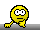## Are we loosers? [🇷 for BE/BA]

Hi ElMaestro,

❝ The problem is not that X changes dimensions, X is the design matrix and this is generally never a square matrix, just full column rank with as many rows as observations. X does not change at all during the optimisation.

Ah, yes!

❝ The issue here is that the determinant suddenly becomes apparently negative.

Might point towards a similar issue in PHX complaining about negative variance components and SAS forcing them to zero.

❝ This is mysterious to me at this point, I am not much into matrices.

So am I. Sorry.

❝ I take it as a case of NM taking some unfortunate decision, which is then corrected afterwards.

Luckily.

❝ However this does give food for thought: if the parameter vector that gives a negative determinant is chosen as a starting value then NM will stop immediately and throw an error - you can try it with the vector above.

❝ Therefore, it may be desirable to ascertain positiveness of that determinant (and the others?) when starting the algo, and to slightly modify individual starter values in case of such suboptimally chosen starter values until Obj.F12 actually returns something meaningful.

But how?

❝ I believe the issue can not be remedied by fiddling with X.

Agree.

❝ Update 8 hours later: …

Sounds reasonable.
But I think that we are going in circles. This story is all about RSABE where we have to go for ABE if swR <0.294 (or the mixed-model for ABE in general). The scaling part is easily doable in R. Since you found a solution for estimating swR and the point estimate, fine. However, if we have to go for ABE, we are still at a loss. Remember:

PROC MIXED   CLASSES SEQ SUBJ PER TRT;   MODEL Y = SEQ PER TRT/ DDFM=SATTERTH;   RANDOM TRT/TYPE=FA0(2) SUB=SUBJ G;   REPEATED/GRP=TRT SUB=SUBJ;   ESTIMATE 'T vs. R' TRT 1 -1/CL ALPHA=0.1; run;For the imbalanced dataset of this post PHX gives me:
Δ 0.03084859
SEΔ 0.03256626
df 18.24154
and therefore, $$\small{CI=\exp (\Delta\mp t_{0.05,df}\times SE)=\left \{ 0.9747, 1.0912 \right \}}$$.

Dif-tor heh smusma 🖖🏼 Довге життя Україна!Helmut SchützThe quality of responses received is directly proportional to the quality of the question asked. 🚮
Science QuotesIng. Helmut Schütz